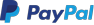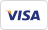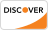R is a programming language is widely used by data scientists and major corporations like Google, Airbnb, Facebook etc. for data analysis. This is a complete ebook on R for beginners and covers basics to advance topics like machine learning algorithm, linear regression, time series, statistical inference etc.

## Key Highlights of R Programming Tutorial PDF are

• 383+ pages
• eBook Designed for beginners
• Beautifully annotated screenshots
• You will get lifetime access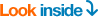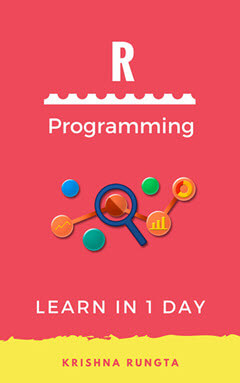### Inside this PDF

1. What is R Programming? Introduction Basics
2. How to Download and Install R, RStudio, and Anaconda on Mac/Windows
3. R Data Types & Operator
4. R Matrix Tutorial: Create,Print,add Column,Slice
5. What is Factor in R? Categorical & Continuous
6. R Data Frames: Create,Append,Select,Subset
7. Lists in R: Create, Select [Example]
8. Sort a data frame using Order
10. Merge data Frames in R:Full and Partial Match
11. Function in R Programming [With Example]
12. IF,ELSE,ELIF, Statement in R
13. For loop in R with Examples for List and Matrix
14. While loop in R with Example
15. apply(),sapply(),tapply() in R with Example
16. Import data into R: Read CSV,Excel,SPSS,Stata,SAS Files
17. Replace missing Values(NA)in R
18. R Exporting Data to CSV, Excel, SAS, STATA, and Text File
19. Pearson, Spearman Correlation in R and Correlation Matrix
20. R Aggregate Function: Summarise & Group_by() [Example]
21. R Select(), Filter(), Arrange(), Pipeline [Example]
22. Scatter plot with ggplot2 [Examples]
23. How to make Boxplot in R (with EXAMPLE)
24. Bar Chart & Histogram in R [Examples]
25. T-Test in R: One-Sample and Paired [Example]
26. R ANOVA Tutorial: One-way & Two-way [with Examples]
27. R Simple, Multiple Linear and Stepwise Regression [with Example]
28. Decision trees in R with Example
29. R Random Forest Tutorial [Examples]
30. Generalized Linear Model (GLM) in R with Example
31. K-means clustering in R with Example
32. R Vs Python: What’s the difference?